# Example Problem Solution

[Page 365]

The following example will illustrate CPM/PERT network analysis and probability analysis.

#### Problem Statement

Given the following AON network and activity time estimates, determine the expected project completion time and variance, as well as the probability that the project will be completed in 28 days or less: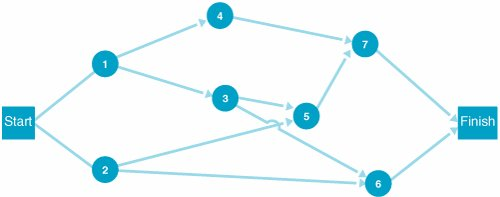Time Estimates (weeks)

Activity

a

m

b

1

5

8

17

2

7

10

13

3

3

5

7

4

1

3

5

5

4

6

8

6

3

3

3

7

3

4

5

#### Solution

Step  1.
Compute the Expected Activity Times and Variances

Using the following formulas, compute the expected time and variance for each activity: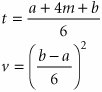[Page 366]
For example, the expected time and variance for activity 1 is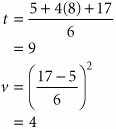These values and the remaining expected times and variances for each activity follow:

Activity

t

v

1

9

4

2

10

1

3

5

4/9

4

3

4/9

5

6

4/9

6

3

7

4

1/9

Step  2.
Determine the Earliest and Latest Times at Each Node

The earliest and latest activity times and the activity slack are shown on the following network: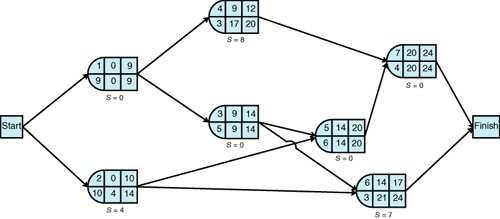[Page 367]
Step  3.
Identify the Critical Path and Compute Expected Project Completion Time and Variance

After observing the foregoing network and those activities with no slack (i.e., S = 0), we can identify the critical path as 1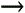357. The expected project completion time ( t P ) is 24 days. The variance is computed by summing the variances for the activities in the critical path: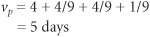Step  4.
Determine the Probability That the Project Will Be Completed in 28 Days or Less

The following normal probability distribution describes the probability analysis: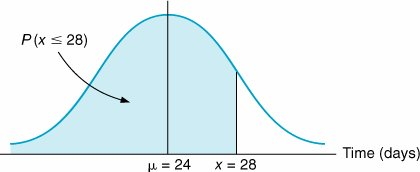Compute Z by using the following formula: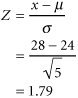The corresponding probability from Table A.1 in Appendix A is .4633; thus,

P ( x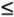28) = .9633Introduction to Management Science (10th Edition)
ISBN: 0136064361
EAN: 2147483647
Year: 2006
Pages: 358

Similar book on Amazon

flylib.com © 2008-2017.
If you may any questions please contact us: flylib@qtcs.net